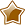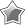# Simple Data Types

## Python Simple Data Types

Python stores different types of information in different data types.

### Strings

These are used to store mixed text, passwords, sentences.

Examples:

• dog
• passw54£\$%^123
• The cat sat on the mat

### Integers

These are used to store whole numbers.

example:

• 435
• -323442

### Floats

These are used to store numbers with a decimal place values.

• 4.13234
• 0.00000000023

## Boolean

These are used to store True or False values

Examples:

• True
• False

## Video 1

Click in the top right of the video to view full screen

## Basic Example

Click in the top right of the video to view full screen

## Challenge 9

Challenge 9 – Hows the temperature?Bronze

• Ask the user the temperature and convert the temperature to an integer.
• If the temperature is above 30 degrees, tell the user it is hot todaySilver

• If the temperature is below 5 degrees, tell the user the it is cold.
• Use the os.system(‘clear’) function to add system clears to your program.Gold

• If the user enters any amount outside of the valid range ( – 50c ./ + 50c) , they should be told that they have entered an invalid value.

## 10

Challenge 10 – Calculate the area of a rectangle.Bronze

• Ask the user to enter the length and width of a rectangle.
• Convert the inputs to integers.
• Output the area of the rectangle.Silver

• Use the .strip() method to remove spaces before and after the user’s input.
• Also calculate the circumference of the rectangle and output the circumference as well.Gold

• Ask the user to enter the lengths and widths of 2 rectangles.
• Calculate the area of both and output which rectangle is higher.

## 11

Challenge 11 – Calculate the missing triangle angle

The angles of a triangle always add up to 180 degrees.Bronze

• Ask the user to enter 2 angles of a triangle and convert the values to integers
• Work out the third angle and output it to the user.Silver

Check each of the values entered to ensure that they are valid ( less than 180 degrees in total) and print out an appropriate response if they don’t enter a valid amount.Gold

• Check the values entered and calculated to work out of the triangle if the triangle is either:
• Equilateral  – All angles equal size.
• Isosceles – Two angles equal size.
• Scalene – All angles of different size.
• Print out an appropriate amount.

## 12

Challenge 12 – Reaction Timer Game

Create a program that tests the user’s reaction time.

*** To complete this challenge you need to watch the advanced tutorial ***Bronze

• Get the program to wait a random amount of seconds  and then ask the user to press enter.Silver

• Record the time the message was displayed and the time the user pressed enter. Work out the response time and print it out to the user.
• use the round() function to convert the user’s float to 2 decimal places.Gold

• If the user took less the 0.1 seconds, tell them well done.
• If the user took longer than 0.5 seconds, tell them their reaction time is too slow.